## QUANTUM & OPTICAL TECHNOLOGY

A. A. Pushkina, G. Maltese, J. I. Costa-Filho, P. Patel and A. I. Lvovsky

#### Superresolution Linear Optical Imaging in the Far Field

Physical Review Letters 127, 253602 (2021)

Stepan Makarenko, Dmitry Sorokin, Alexander Ulanov and A. I. Lvovsky

#### Aligning an optical interferometer with beam divergence control and continuous action space

arXiv:2107.04457

Arsen Kuzhamuratov, Dmitry Sorokin, Alexander Ulanov and A. I. Lvovsky

arXiv:2107.03741

D.A. Chermoshentsev, A.O. Malyshev, E.S. Tiunov, D. Mendoza, A. Aspuru-Guzik, A.K. Fedorov and A.I. Lvovsky

#### Polynomial unconstrained binary optimisation inspired by optical simulation

arXiv:2106.13167

D. Beloborodov, A. E. Ulanov, J. Foerster, S. Whiteson and A. I. Lvovsky

#### Reinforcement learning enhanced quantum-inspired algorithm for combinatorial optimization

Machine Learning: Science and Technology, Volume 2, 025009 (2021)

Xianxin Guo, Thomas D. Barrett, Zhiming M. Wang and A.I. Lvovsky

#### Backpropagation through nonlinear units for the all-optical training of neural networks

Photonics Research 9, B71-B80 (2021)

D. Drahi, D. V. Sychev, K. K. Pirov, E. A. Sazhina, V. A. Novikov, I. A. Walmsley and A. I. Lvovsky

#### Entangled resource for interfacing single- and dual-rail optical qubits

Quantum 5, 416 (2021)

## A homodyne tomography tutorial

How can one determine a quantum state’s Wigner function? Although it cannot be measured directly as a probability density, all its marginal distributions can. Once we know all the marginal distributions associated with different quadratures – i.e. the Wigner function projections upon various vertical planes – we can reconstruct the Wigner function. This reconstruction procedure is very similar to that used by medical doctors in computer tomography – where one reconstructs a three-dimensional picture of a tissue anomaly from a set of two-dimensional X-ray images acquired from different angles.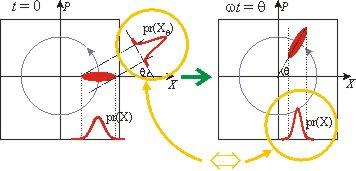Fig. 1

So our goal is to determine the marginal distributions for all different “points of view”. Suppose we can measure the coordinate of our oscillator (for example, by taking its photograph at a given time). Repeating this procedure many times for a set of identical quantum states we obtain the marginal distribution pr(X) for quadrature X. This is, however, insufficient for tomographic reconstruction of the Wigner function: we need distributions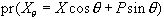for all values of q. In order to understand how to obtain these distributions we need to recall that our oscillator, well, oscillates. That is, our Wigner function rotates around the phase space origin with the oscillation frequency w. The marginal distribution pr(X) measured at the moment t = q / w is the same as the marginal distribution pr(Xq) measured at the moment t = 0. Therefore, instead of measuring the marginal distributions at a particular moment for all “view angles” we can measure marginal distribution associated with one view angle, but at different moments [Fig. 1].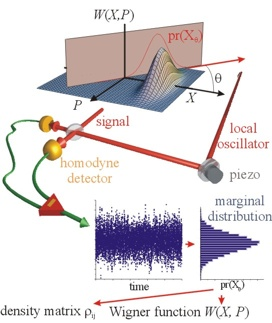Fig. 2

Let us now remember that we work with light, not with mechanical oscillators. As we know from classical physics, these two physical systems are described by the same equations of motion. The role of the mechanical coordinate in the electromagnetic wave is played by the electric field. If we had an “electroscope” able to perform phase-sensitive measurements of the electric field in an electromagnetic mode, we would be able to reconstruct the “quantum portrait” of the mode’s quantum state – its Wigner function.

Unfortunately, such an “electroscope” does not exist. Typical oscillation frequencies of the light fields are hundreds of terahertz, and no one can build a detector that can follow such fast changes. Yet one can use a trick that allows to do phase-sensitive measurements of the electric field using regular, “slow” detectors. This trick is called balanced homodyne detection – here is its main idea.

Quantum objects are usually small, and electromagnetic fields that exhibit quantum properties are usually weak. Let us overlap the mode whose quantum properties we want to measure (the “signal” mode) with a relatively strong laser beam (the “local oscillator”) on a beam splitter [fig. 2]. If the electric fields in the two modes are Es and ELO, the fields emerging in the two beam splitter output ports are given by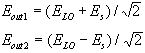Let us now have both beam coming out of the beam splitter hit high-efficiency photodiodes. A photodiode is a device which generates electric current proportional to the intensity (not the amplitude) of the electromagnetic field incident on it. Our two photodiodes produce photocurrents that we subtract from each other. The photocurrent difference is given by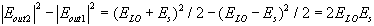since ELO >> Es, we neglect the quantum noise of the local oscillator field and find out that the photocurrent difference is proportional to the amplitude of the signal field – exactly the quantity we are looking for! By changing the relative optical phase of the local oscillator and signal waves, we measure the electric field at different phases. At each phase, we perform a multitude of electric field measurements (each time preparing an identical quantum state in the signal channel) thus obtaining the marginal distribution. A set of marginal distributions for various q’s will provide us with full information about the quantum state – and allows us to reconstruct its Wigner function and the density matrix.

An excellent introduction into nonclassical light and methods of its characterization is given in the textbook by U. Leonhardt “Measuring the quantum state of light”, CambridgeUniversity Press, 1997.

Our review paper on homodyne tomography

Gerd Breitenbach’s homodyne tomography page

## Vacuum state

This is the simplest quantum state altogether: no light! But even when we switch off the light, the uncertainty principle holds valid, so both position and momentum exhibit some fluctuations. These are the vacuum fluctuations and they are the same for X and P – so the Wigner function is a round hill centered at the origin of phase space.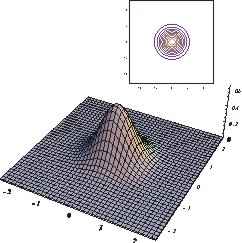## Coherent state

This state is emitted by a laser. Its Wigner function is the same as for the vacuum state, but displaced in the phase space so there is a nonzero average for the electric field.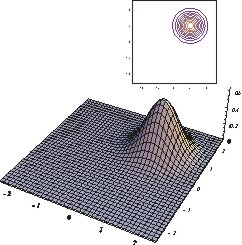## Thermal state

This is the state of radiation a heated black body emits. It has no phase and its photon statistics is that of Bose-Einstein. By room temperature the thermal state is to a good approximation vacuum.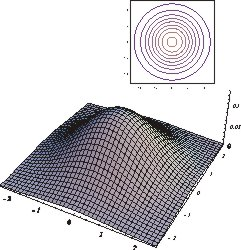## Squeezed vacuum state

The first nonclassical state on this page. The uncertainty in one quadrature is reduced at the other quadrature’s expense. The product of the two is however the same as for the vacuum: this is a minimum uncertainty state.
See our experimental work on generating squeezed light.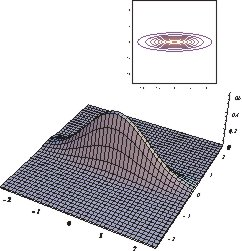## Single-photon Fock state

The name says it all – a state containing exactly one photon. We did an experimental project on this one, too!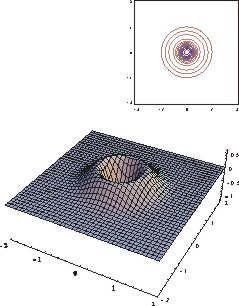## Four-photon Fock state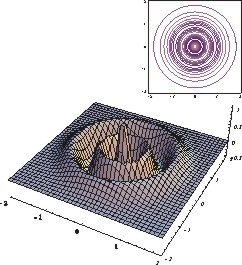## Schrödinger cat state

This is a coherent superposition of two coherent states: |a> and |-a>. It is hard to generate experimentally, but the Wigner function is instructive to look at. We see two round hills at the top and at the bottom, associated with each coherent state involved. If our ensemble were an incoherent, statistical mixture of these two states, its Wigner function would just feature these two hills. But because the superposition is coherent, the Wigner function exhibits an additional, highly nonclassical feature in the middle: a fine structured interference pattern with negative regions.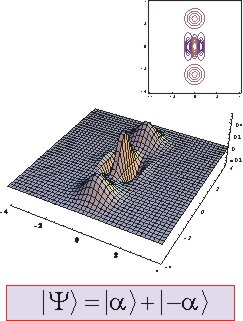## Star state

This state is an analog of the squeezed state, but is generated (in theoreticians’ notebooks) by means of 3-photon, rather than 2-photon, down-conversion.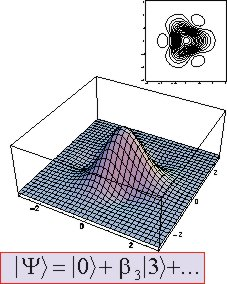## Squeezed single-photon Fock state

A limiting case of the “odd” Schrödinger cat state |α> – |-α> for small α.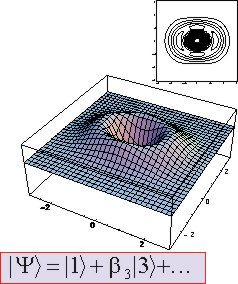Gerd Breitenbach’s page featuring another gallery of quantum states.

## Storage of light via electromagnetically-induced transparency

This tutorial gives a technical description of the light storage phenomenon. The physical description – i.e. not what happens, but why it happens, is much more involved and is hard to describe in the semi-popular language.

Consider a glass cell filled with a gas of so-called Lambda-type atoms – a three-level system with two ground and one excited states: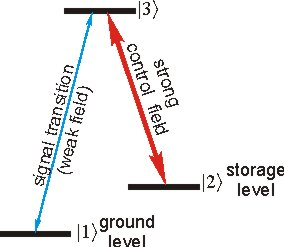We assume the gas to be optically dense on the transition between levels 1 and 3, that is, a laser beam of the transition wavelength will be absorbed by the gas. If we now, at the same time, apply an additional, strong laser field to the transition 2 – 3 to exhibit a narrow transparency window in the absorption spectrum. Moreover, if the blue laser is pulsed, the pulse will propagate through the cell at a speed much less than the vacuum speed of light – possibly slower than a bicycle! This phenomenon is called electromagnetically-induced transparency (EIT).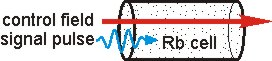Let us now turn the control (red) laser off slowly while the signal (blue) pulse is entirely within the sample making its way through. As we reduce the control field, the signal pulse will propagate slower and slower and will eventually stop. The quantum information contained in the pulse will be transferred to the atoms – more precisely, to the coherent superposition of the two ground levels 1 and 2. Because both are low energy states, their superposition is long-lived, that is – subject to little decay. It can remain unchanged for hundreds of microseconds – an epoch on the atomic time scale!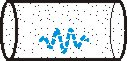Theoreticians say, this storage procedure is completely reversible. When we wish to read our memory, we slowly turn the control field back on. The signal pulse will be reemitted with its original shape, phase and in its original quantum state – thus completing the storage/release procedure.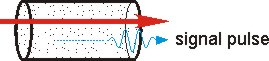Here is a little movie showing the process of light storage. Notice how the EIT absorption and dispersion, as well as the group velocity of the light pulse change as a function of the control field.

## A Wigner function tutorial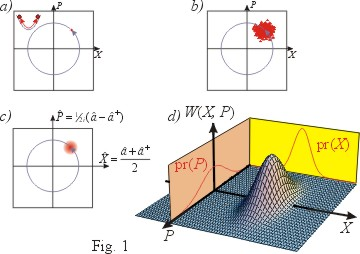Consider a classical harmonic oscillator. Its motion can be completely described by a point in the phase space – the two-dimensional space with the particle’s coordinate X and momentum P as dimensions (quadratures) [Fig. 1(a)]. For a large number of identical classical oscillators, one can talk about the phase-space probability distribution – a function W(X, P) which indicates the probability of finding a particle at a certain point in the phase space [Fig. 1(b)]. This function must, of course, be non-negative and normalized: its integral over the entire phase space must be equal to one.   This classical probability distribution has another important property. Consider a series of measurements in which we only measure the oscillator’s coordinate but not the momentum. After a large number of such measurements, one obtains the probability distribution associated with the coordinate – we call this a marginal distribution pr(X). This distribution is related to the phase-space probability density in the following way: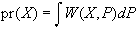In other words, a marginal distribution is just a density projection of W(X, P) onto a plane associated with the given quadrature [Fig 1(d)].

In the quantum world [Fig. 1(c)], the notion of a “certain point in the phase space” does not make sense because the position and the momentum cannot be measured simultaneously (Heisenberg’s uncertainty principle). Neither does the phase-space probability density. However, even in the quantum domain one can perform quantum measurements of a single quadrature – be it X, P, or their linear combination. A multiple measurement of a quadrature on a set of identical quantum states will yield a probability density associated with this quadrature, i.e. a marginal distribution. Is there any connection between marginal distributions for different quadratures?

In the classical world this connection exists – through the phase-space probability density as discussed above. The amazing fact is that even in the quantum domain there exists so called phase space quasiprobability density – called the Wigner function – with exactly the same property. A marginal distribution associated with a particular quantum state and a particular quadrature is a projection of the state’s Wigner function upon the relevant vertical plane.

The Wigner function of a given state can be calculated from its density matrix. For each quantum ensemble there exists a Wigner function. Just as the classical phase-space probability density, it is real and normalized. However, the Wigned function has one very important difference from its classical analogue. Because by itself it does not have a meaning of a probability density, it does not have to be positive definite. An example is provided by so-called Fock states of harmonic oscillators – the states of definite energy. No matter what the energy is, the phase space has regions where the Wigner function takes on negative values.